﻿ 基于CFD的船舶双螺旋桨水动力性能分析
 舰船科学技术2019, Vol. 41Issue (3): 37-41PDF

Analysis for hydrodynamic performance of ship's dual-propeller based on CFD
KONG Jin-ping, WU Bo-tao, KONG Ling-zhi
China Satellite Maritime Tracking and Control Department, Jiangyin 214431, China
Abstract: At present, the ship with dual-propeller widely, but due to the mutual interference between the oars, causing the hydrodynamic performance of the propeller are different. In this paper, the three-dimensional model of dual-propeller would build by ICEM software and sliding mesh. The Fluent software was used to predict the hydrodynamic performance of dual-propeller. The effect on hydrodynamic performance by different turbulent model was analyzed, between k-ε and k-ω. The propulsion efficiency of dual-propeller was higher compare to single propeller by numerical calculation using symmetrical boundary model.
Key words: CFD     hydrodynamic performance     dual-propeller     sliding mesh
0 引　言

1 数学模型

1.1 控制模型

CFD的基本方程有质量守恒方程、动量方程和能量方程，分为积分形式和微分形式2种。积分形式是流体各种物理量之间的积分关系式，求解积分形式基本方程可以得到总体性能关系，如流体与物体之间的总作用力和总能量交换等。微分形式是流体各种物理量之间的微分关系式，求解微分形式基本方程可以得到流场内部的一些细节，包括各网格点上流体的速度、压力和温度等。

1）质量守恒方程

 $\int_{V}{\frac{\partial \rho }{\partial t}\delta V}+\oint_{S}{\rho v \cdot n\delta S}=0\text{。}$ (1)

2）动量方程

 $\rho \frac{{\rm d}v}{{\rm d}t}=\rho f+Div\sigma \text{。}$ (2)

3）能量方程

 $\begin{split} & \displaystyle\frac{\partial }{{\partial t}}\left[ {\rho \left( {\displaystyle\frac{{{v^2}}}{2} + e} \right)} \right] + \nabla \cdot \left[ {\rho v\left( {\displaystyle\frac{{{v^2}}}{2} + e} \right)} \right] = \\ & \rho f \cdot v + \rho {q_k} + \nabla \cdot \left( {\sigma \cdot v} \right) + \nabla \cdot \left( {k\nabla t} \right)\text{。} \end{split}$ (3)

 $\sigma =-pI+\left( {\mu }'-\frac{2}{3}\mu \right)\left( \nabla \cdot v \right)I+2\mu S\sigma \text{。}$

 $\frac{{{\rm d}V}}{{{\rm d}t}} = f - \frac{1}{\rho }\nabla p + \frac{1}{\rho }Div\tau \text{，}$ (4)
 $\begin{split} & {\displaystyle\frac{\partial }{{\partial t}}\left[ {\rho \left( {\displaystyle\frac{{{v^2}}}{2} + e} \right)} \right] + \nabla \cdot \left[ {\rho v\left( {\displaystyle\frac{{{v^2}}}{2} + e} \right)} \right]}=\\ & { \rho f \cdot v + \rho {q_k} - \nabla \cdot\left( {pV} \right) + \rho \Phi + \nabla \cdot \left( {k\nabla T} \right) + v \cdot Div\sigma }\text{。} \end{split}$ (5)

1.2 湍流模型

1.3 滑动网格

2 计算模型

CFD计算工具采用主流分析软件Fluent，流体介质为水，螺旋桨区域采用相对运动的网格边界。根据模型特点，CFD计算采用边界条件类型包含：速度进口、对称面、壁面、interface、远场边界等。受螺旋桨限制，速度进口均采用来流速度为14 kn（即7.196 m/s），远场即为自由出流边界。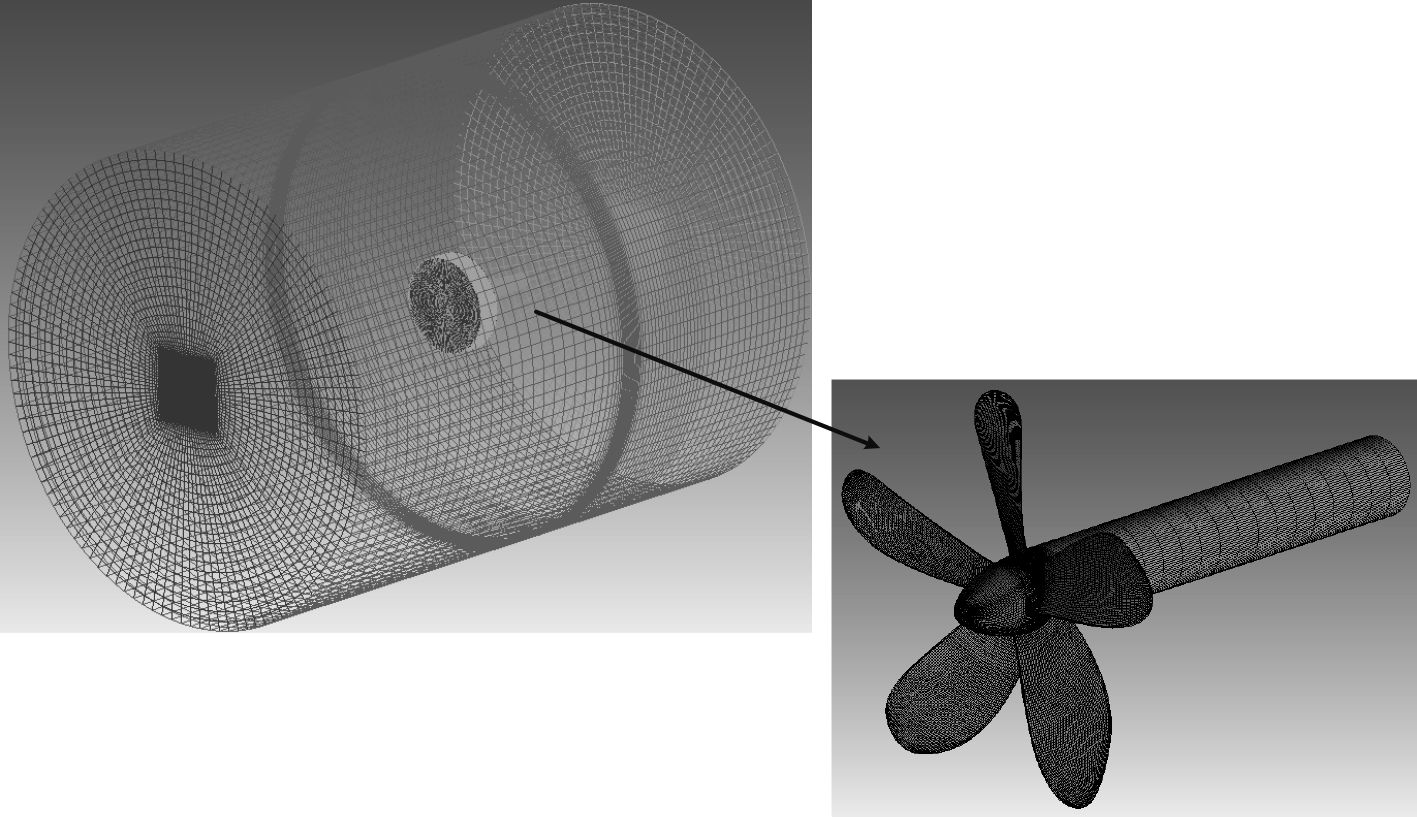图 1 模型网格示意 Fig. 1 Model grid schematic
3 数值分析 3.1 收敛判据

CFD数值计算时边界条件的设置主要有速度进口边界条件、对称面边界条件、壁面边界条件、interface边界条件和边界条件，其中远场的边界条件主要根据来流情况设置。对模型的CFD计算过程都较为顺畅，5 000步左右趋于收敛，如图2所示。最后残差收敛到10–5以下，管道进出口流量差小于0.5%，这样可以判定结果已收敛完全。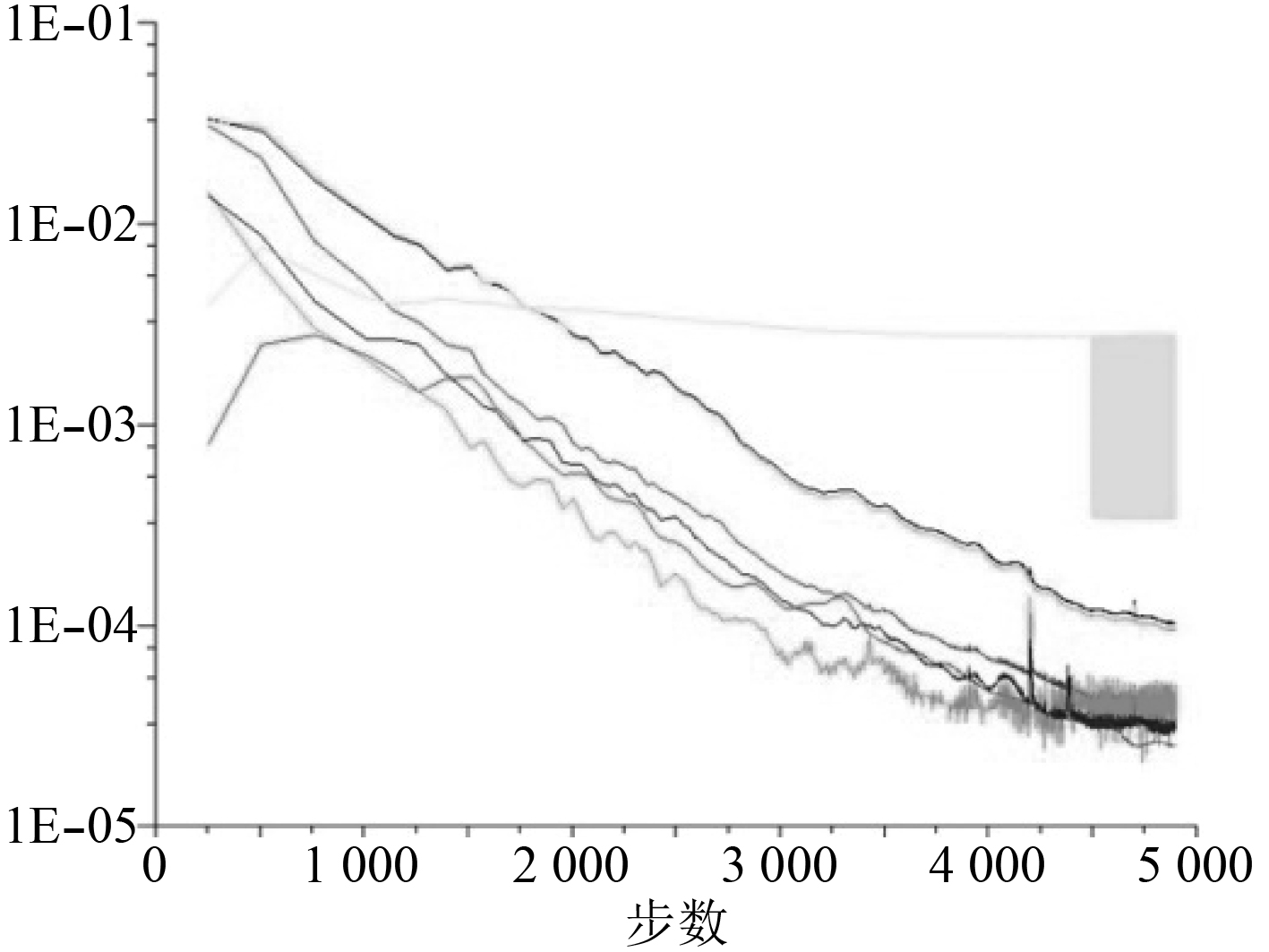图 2 残差曲线示意图 Fig. 2 Schematic diagram of residual curve
3.2 不同湍流模型数值分析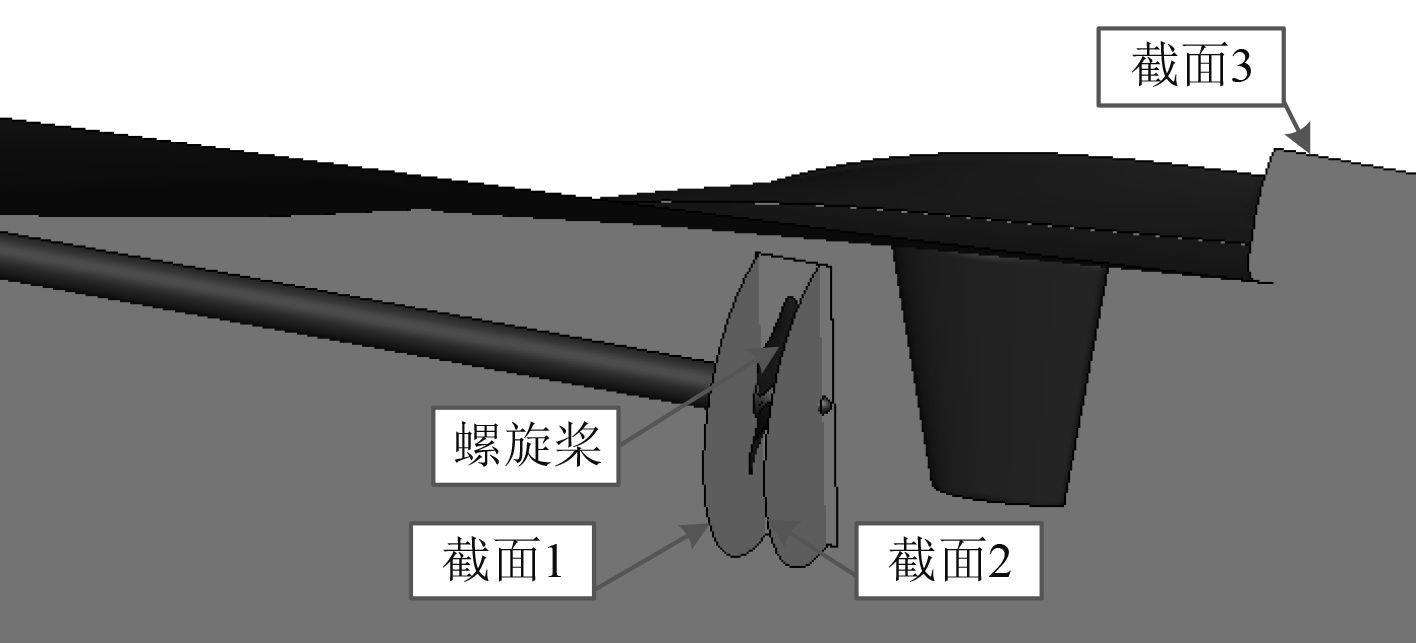图 3 流场剖面示意图 Fig. 3 Schematic diagram of Flow profile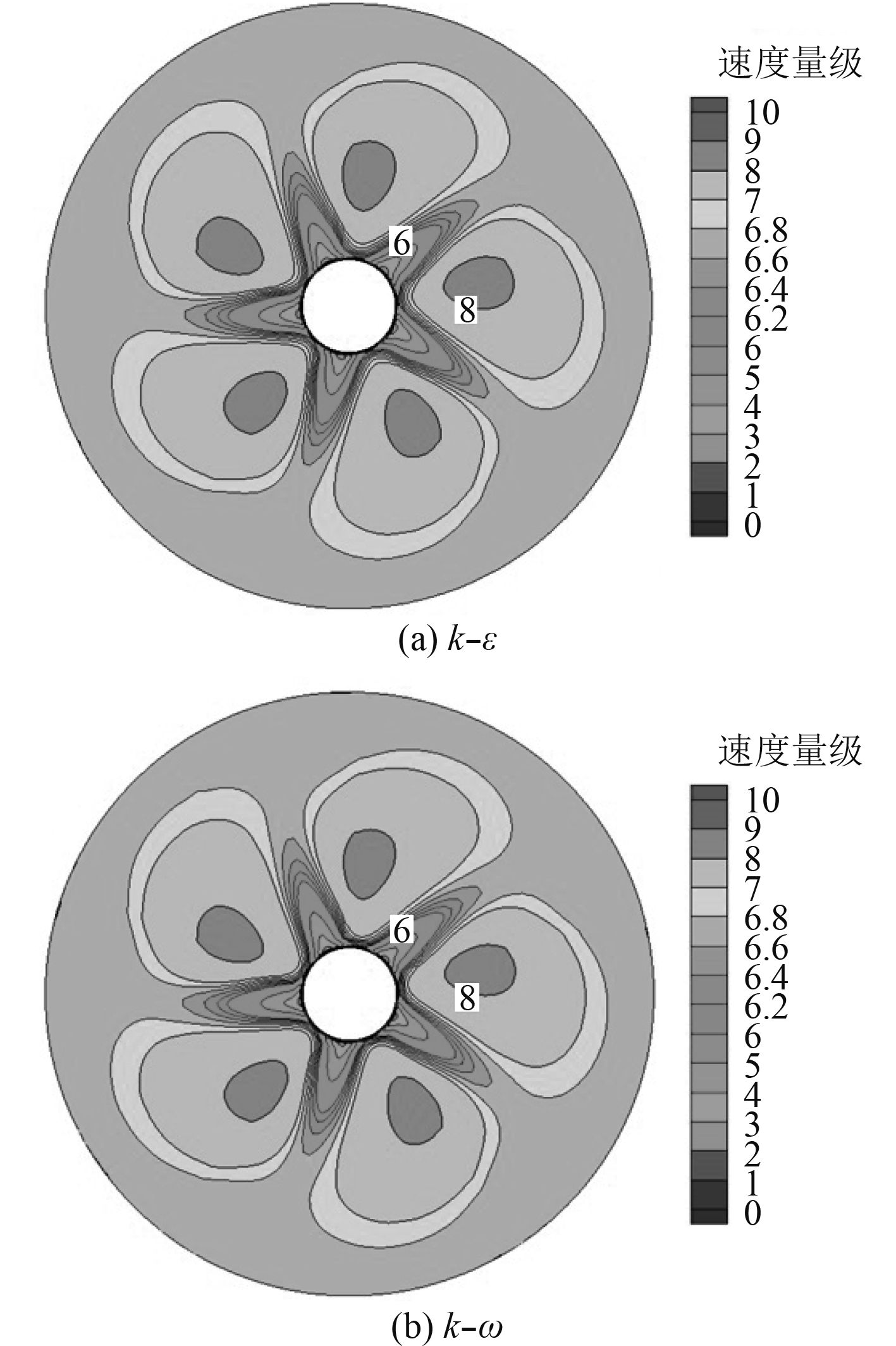图 4 截面1速度场分布 Fig. 4 Velocity field distribution of section 1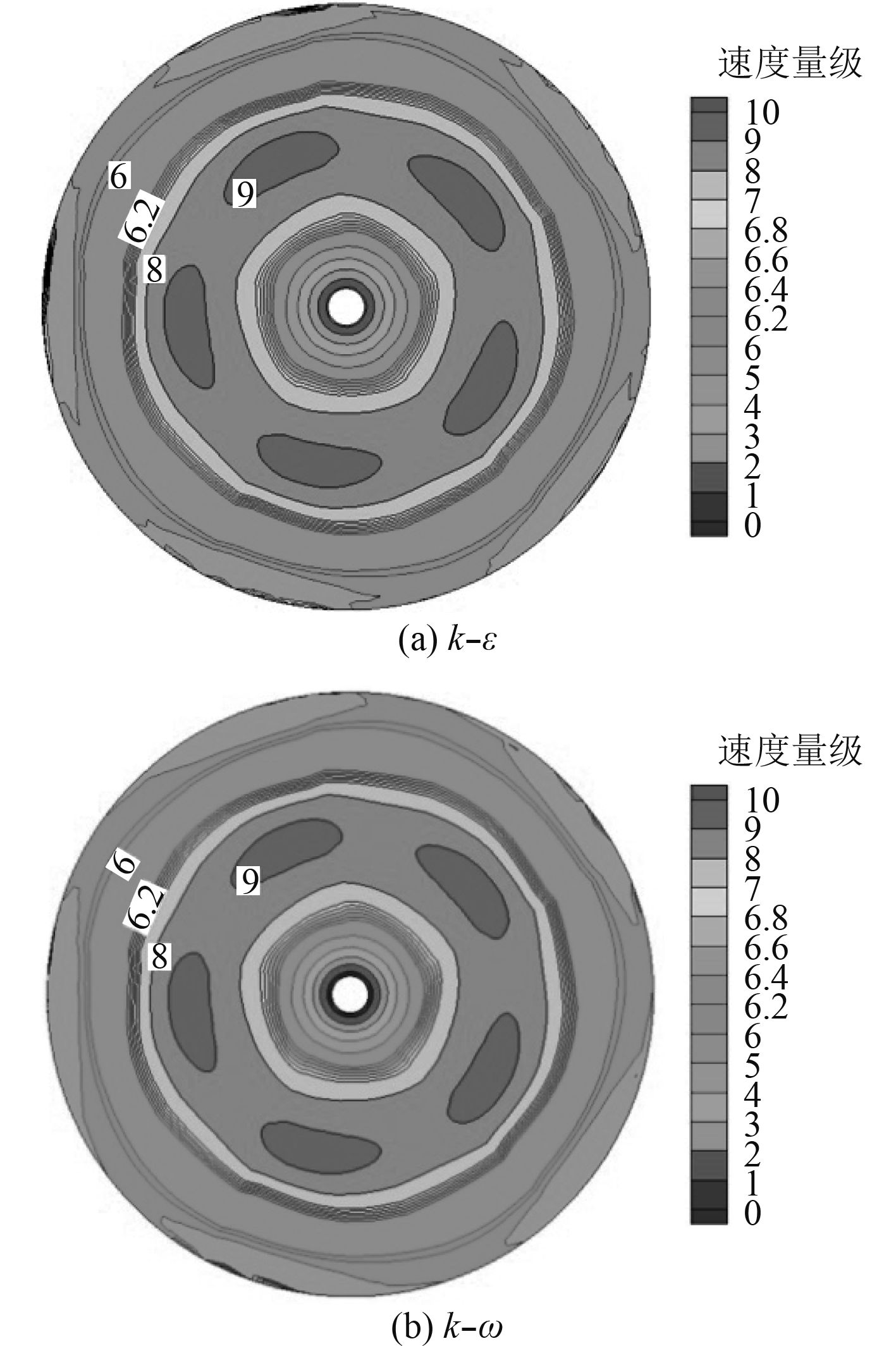图 5 截面2速度场分布 Fig. 5 Velocity field distribution of section 2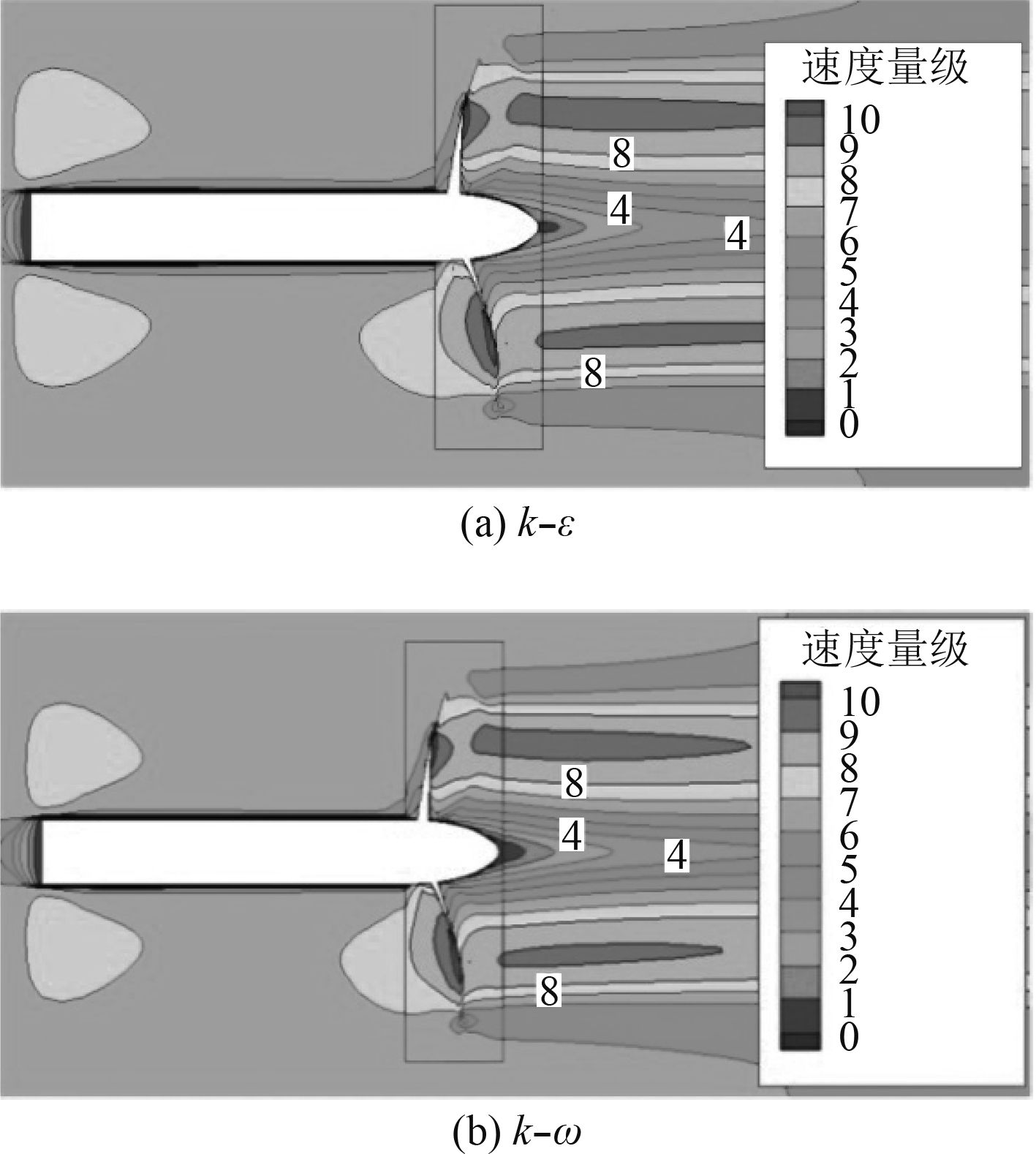图 6 截面3速度场分布 Fig. 6 Velocity field distribution of section 3表 1 不同湍流模型螺旋桨效率 Tab.1 Propeller's efficiency of different turbulence model
3.3 双螺旋桨数值分析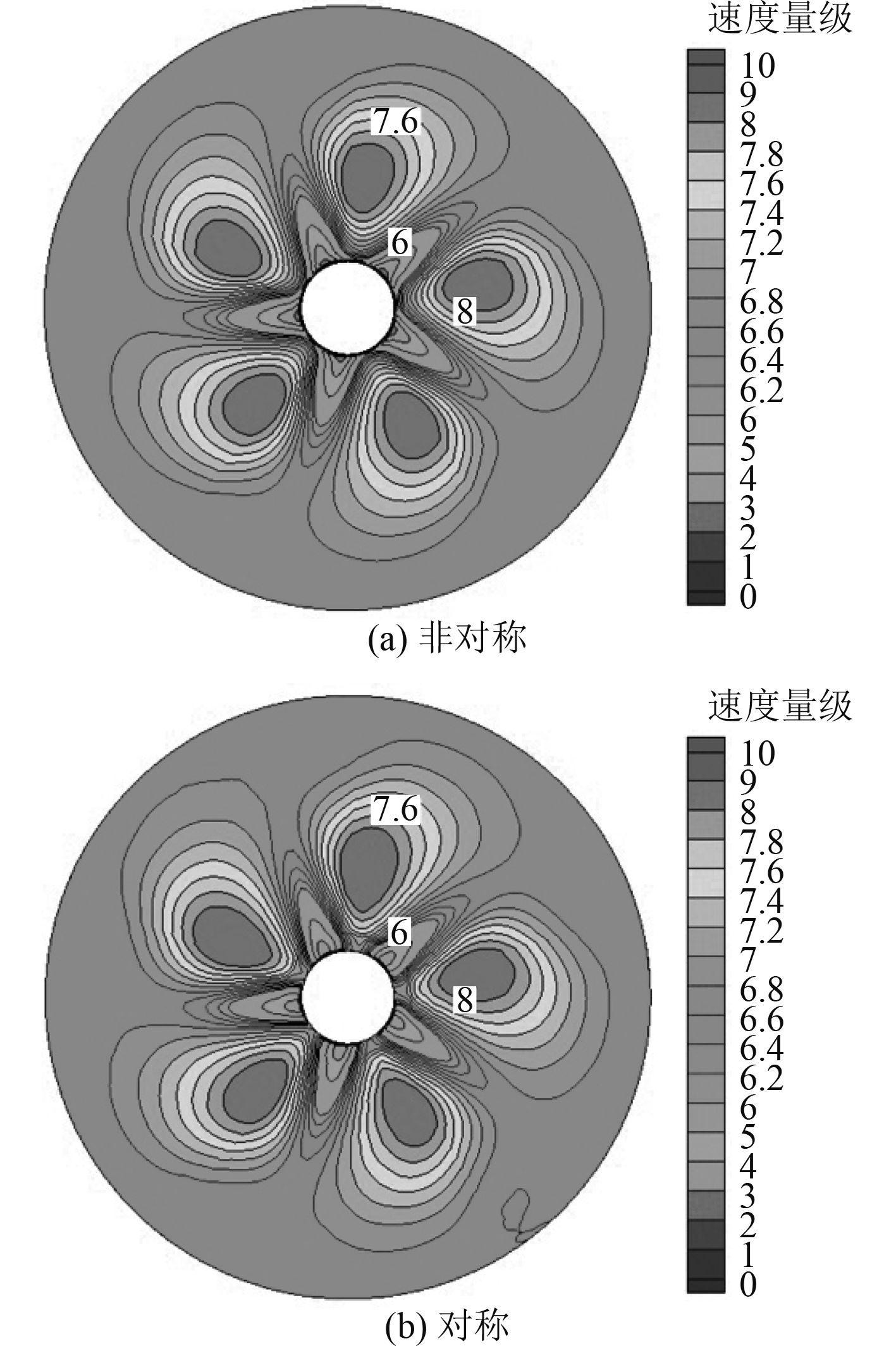图 7 截面1速度场分布 Fig. 7 Velocity field distribution of section 1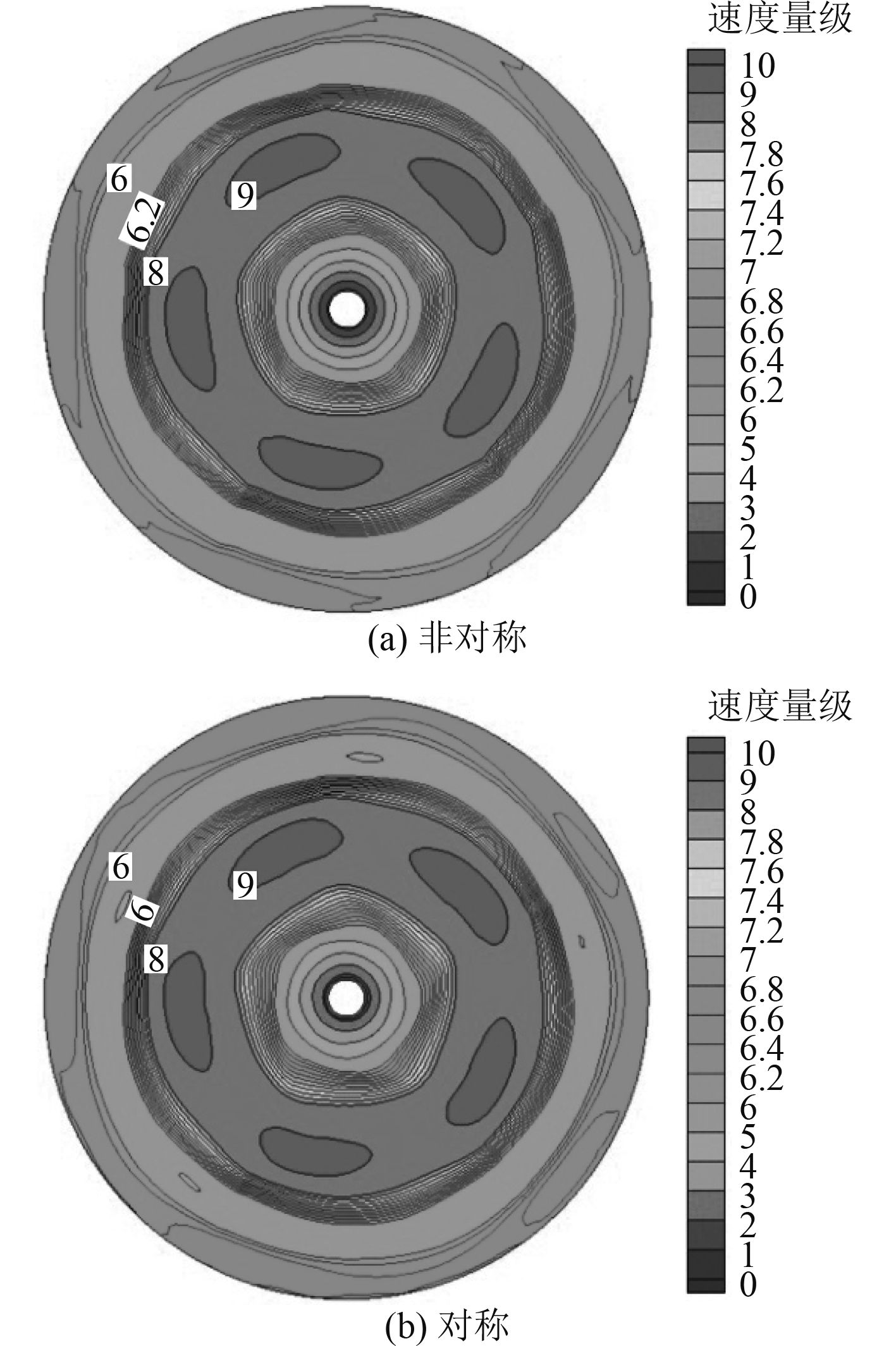图 8 截面2速度场分布 Fig. 8 Velocity field distribution of section 2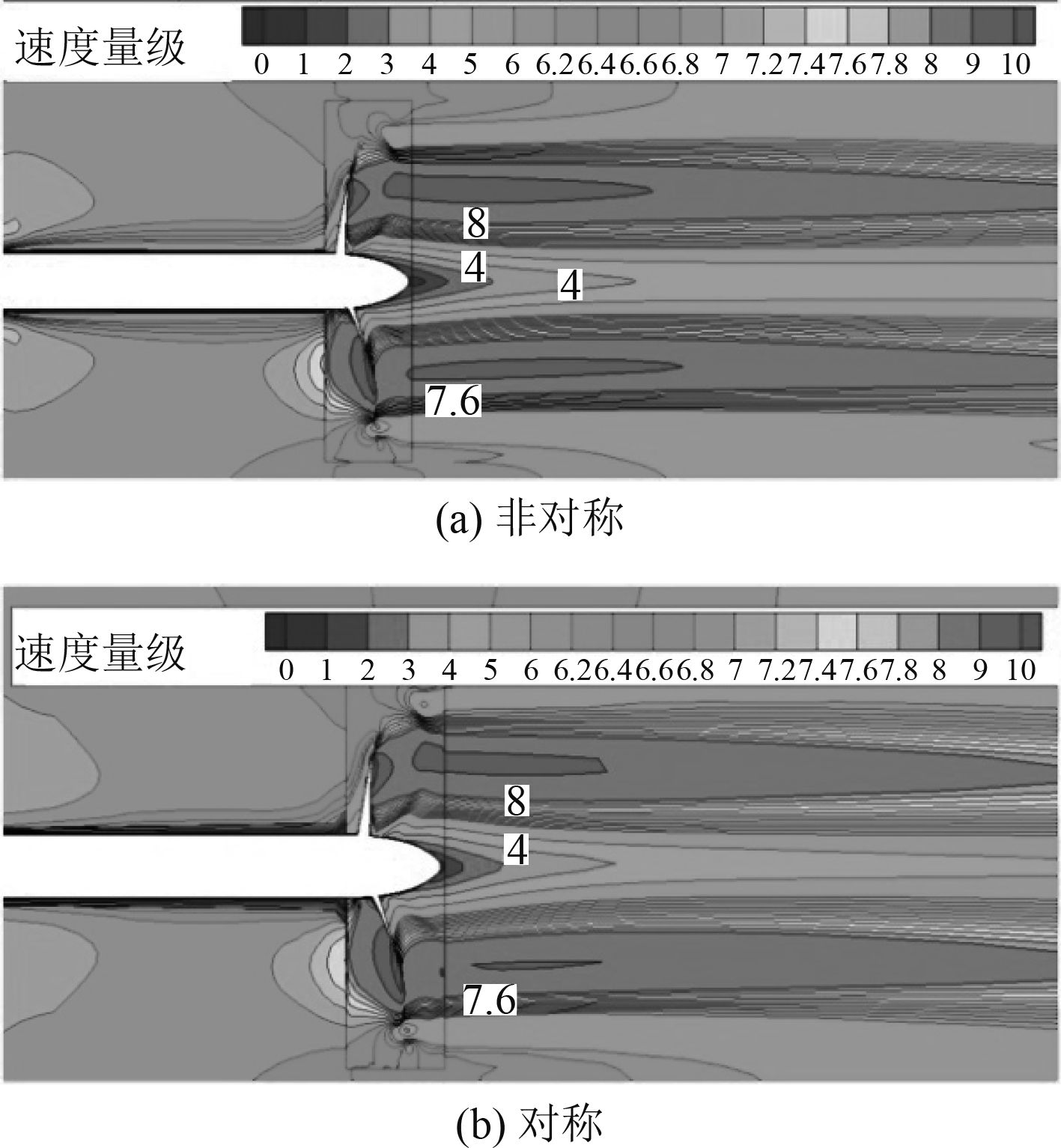图 9 截面3速度场分布 Fig. 9 Velocity field distribution of section 3表 2 双螺旋桨效率 Tab.2 Dual-propeller's efficiency
4 结　语

  FRANCISCO P, FRANCESCO S. Experiment and numerical investigation of the cavitation pattern on a marine propeller[C]//24th Symposium on Naval Hydrodynamics, 2002.  WANG Chao, HUANG Sheng, CHANG Xin, et al. Application of periodic boundary condition on the propeller's open water performance[J]. Journal of Marine Science and Application, 2010, 9(3): 262-267. DOI:10.1007/s11804-010-1005-6  MARIO F, GIULIO G, ROBERTO C. Effect of the number of blades on propeller wake evolution[J]. Exp Fluids, 2008, 44: 409-418. DOI:10.1007/s00348-007-0385-0  张漫, 黎胜. 基于滑移网格技术计算螺旋桨水动力性能研究[J]. 船海工程, 2013, 42(5). ZHANG Man, LI Sheng. Based on sliding gird to calculate the hydrodynamic performance of propeller[J]. Ship Ocean Engineering, 2013, 42(5).  李卉, 邱磊. 螺旋桨水动力性能研究进展[J]. 舰船科学技术, 2011, 33(12): 3-8. LI Hui, QIU Lei. Development and present situation of the propeller hydrodynamic performance[J]. Ship Science and Technology, 2011, 33(12): 3-8. DOI:10.3404/j.issn.1672-7649.2011.12.001  Proceedings of 22nd ITTC Propulsion Committee Propeller RANS/PANEL Method Workshop. Grenoble, France, April, 1998, 5–6.  RHEE S, JOSHI S. CFD validation for a marine propeller using an unstructured mesh based RANS method[C]// Proceedings of FEDSM'03, Honolili, USA, 2003.  MIKKELSEN R, ANDERSEN P, SORENSEN J N. Modeling of behind condition wake flow in RANS computation on a conventional and high skew propeller[C]//Proceedings of 10th Numerical Towing Tank Symposium, Hamburg, Germany, September, 2007.  KRASILNIKOV V, SUN J, HALSE K H. CFD investigation in scale effect on propellers with different magnitude of skew in turbulent flow[C]//First International Symposium on Marine Propulsors SMP'09, Trondheim, Norway, June, 2009  叶剑平, 庄光宇. 螺旋桨水动力性能计算粘性流体CFD方法的应用与研究[J]. 舰船科学技术, 2013, 35(4): 29-34. YE Jian-ping, ZHUANG Guang-yu. Application and study of the computational fluid dynamics method in the computation of a propeller's hydrodynamic performance[J]. Ship Science and Technology, 2013, 35(4): 29-34. DOI:10.3404/j.issn.1672-7649.2013.04.007